## Description

Returning the Chi-squared test of two given variables with count, percentages and Pearson's residuals table.

### Variable description

Two variables specified:

• "gender" ("Gender") with 673 valid values and
• "dwell" ("Dwelling") with 662 valid values.

#### Introduction

Crosstables are applicable to show the frequencies of categorical variables in a matrix form, with a table view.

We will present four types of these crosstables. The first of them shows the exact numbers of the observations, ergo the number of the observations each of the variables' categories commonly have.

The second also shows the possessions each of these cells have, but not the exact numbers of the observations, rather the percentages of them from the total data.

The last two type of the crosstabs contain the so-called row and column percentages which demonstrate us the distribution of the frequencies if we concentrate only on one variable.

After that we present the tests with which we can investigate the possible relationships, associations between the variables, like Chi-squared test, Fisher Exact Test, Goodman and Kruskal's lambda.

In the last part there are some charts presented, with that one can visually observe the distribution of the frequencies.

### Counts

Counted values: "gender" and "dwell"
city small town village Missing Sum
male 338 28 19 25 410
female 234 3 9 17 263
Missing 27 2 2 5 36
Sum 599 33 30 47 709

Most of the cases (338) can be found in "male-city" categories. Row-wise "male" holds the highest number of cases (410) while column-wise "city" has the utmost cases (599).

### Percentages

Total percentages: "gender" and "dwell"
city small town village Missing Sum
male 47.67 3.95 2.68 3.53 57.83
female 33 0.42 1.27 2.4 37.09
Missing 3.81 0.28 0.28 0.71 5.08
Sum 84.49 4.65 4.23 6.63 100
Row percentages: "gender" and "dwell"
city small town village Missing
male 82.44 6.83 4.63 6.1
female 88.97 1.14 3.42 6.46
Missing 75 5.56 5.56 13.89
Sum 84.49 4.65 4.23 6.63
Column percentages: "gender" and "dwell"
city small town village Missing Sum
male 56.43 84.85 63.33 53.19 57.83
female 39.07 9.09 30 36.17 37.09
Missing 4.51 6.06 6.67 10.64 5.08

### Tests of Independence

In the below tests for independece we assume that the row and column variables are independent of each other. If this null hypothesis would be rejected by the tests, then we can say that the assumption must have been wrong, so there is a good chance that the variables are associated.

#### Chi-squared test

One of the most widespread independence test is the Chi-squared test. While using that we have the alternative hypothesis, that two variables have an association between each other, in opposite of the null hypothesis that the variables are independent.

We use the cell frequencies from the crosstables to calculate the test statistic for that. The test statistic is based on the difference between this distribution and a theoretical distribution where the variables are independent of each other. The distribution of this test statistic follows a Chi-square distribution.

The test was invented by Karl Pearson in 1900. It should be noted that the Chi-squared test has the disadvantage that it is sensitive to the sample size.

##### Criteria

Before analyzing the result of the Chi-squared test, we have to check if our data meets some requirements. There are two widely used criteria which have to take into consideration, both of them are related to the so-called expected counts. These expected counts are calculated from the marginal distributions and show how the crosstabs would look like if there were complete independency between the variables. The Chi-squared test calculates how different are the observed cells from the expected ones.

The two criteria are:

• none of the expected cells could be lower than 1
• 80% of the expected cells have to be at least 5

Let's look at on expected values then:

city small town village
male 349 18.91 17.08
female 223 12.09 10.92

We can see that the Chi-squared test met the requirements.

So now check the result of the test:

Pearson's Chi-squared test: table
Test statistic df P value
12.64 2 0.001804 * *

To decide if the null or the alternative hypothesis could be accepted we need to calculate the number of degrees of freedom. The degrees of freedom is easy to calculate, we substract one from the number of the categories of both the row and the coloumn variables and multiply them with each other.

To each degrees of freedom there is denoted a critical value. The result of the Chi-square test have to be lower than that value to be able to accept the nullhypothesis.

It seems that a real association can be pointed out between gender and dwell by the Pearson's Chi-squared test (χ=12.64) at the degree of freedom being 2 at the significance level of 0.001804 * *.

The association between the two variables seems to be weak based on Cramer's V (0.1001).

• Fisher, R. A. (1922): On the interpretation of chi-square from contingency tables, and the calculation of P. Journal of the Royal Statistical Society 85 (1): 87-94.
• Fisher, R.A. (1954): Statistical Methods for Research Workers. Oliver and Boyd.

The residuals show the contribution to reject the null hypothesis at a cell level. An extremely high or low value indicates that the given cell had a major effect on the resulting chi-square, so thus helps understanding the association in the crosstable.

Residuals: "gender" and "dwell"
city small town village
male -3.08 3.43 0.76
female 3.08 -3.43 -0.76

Based on Pearson's residuals the following cells seems interesting (with values higher than 2 or lower than -2):

• "male - city"
• "female - city"
• "male - small town"
• "female - small town"
##### References
• Snedecor, George W. and Cochran, William G. (1989): Statistical Methods. Iowa State University Press.
• Karl Pearson (1900): Philosophical Magazine, Series 5 50 (302): 157-175.

#### Fisher Exact Test

An other test to check the possible association/independence between two variables, is the Fisher exact test. This test is especially useful with small samples, but could be used with bigger datasets as well.

We have the advantage while using the Fisher's over the Chi-square test, that we could get an exact significance value not just a level of it, thus we can have an impression about the power of the test and the association.

The test was invented by, thus named after R.A. Fisher.

The variables seems to be dependent based on Fisher's exact test at the significance level of 0.0008061 * * *.

### Direction of relationship

#### Goodman and Kruskal's lambda

With the help of the Goodman and Kruskal's lambda we can look for not only relationship on its own, which have directions if we set one variable as a predictor and the other as a criterion variable.

The computed value for Goodman and Kruskal's lambda is the same for both directions: 0. For this end, we do not know the direction of the relationship.

### Charts

If one would like to investigate the relationships rather visually than in a crosstable form, there are several possibilities to do that.

###### Heat map

At first we can have a look at on the so-called heat map. This kind of chart uses the same amount of cells and a similar form as the crosstable does, but instead of the numbers there are colours to show which cell contains the most counts (or likewise the highest total percentages).

The darker colour is one cell painted, the most counts/the higher total percentage it has.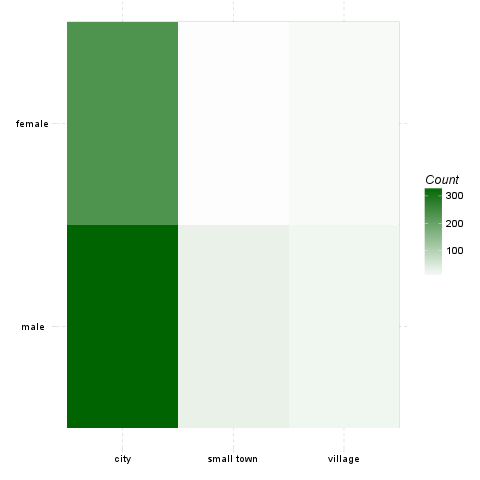There can be also shown the standardized adjusted residual of each cells: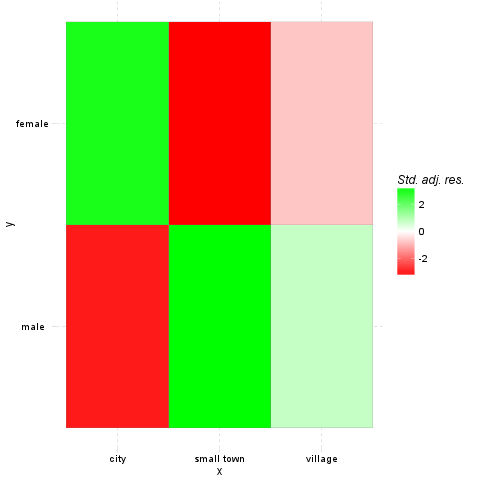###### Mosaic chart

In front of the heat map, on the mosaic charts, not only the colours are important. The size of the cells shows the amount of the counts one cell has.

The width on the axis of gender determinate one side and the height on the axis of the dwell gives the final shape of the box. The box which demonstrates a cell from the hypothetic crosstable. We can see on the top of the chart which category from the dwell draw the boxes what kind of colour.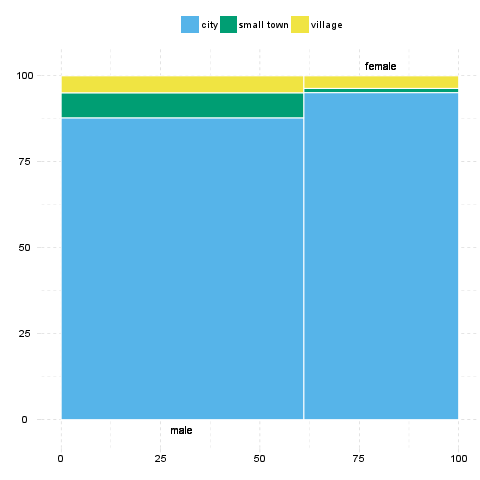###### Fluctuation diagram

At last but not least have a glance on the fluctuation diagram. Unlike the above two charts, here the colours does not have influence on the chart, but the sizes of the boxes, which obviously demonstrates here as well the cells of the crosstable.

The bigger are the boxes the higher are the numbers of the counts/the total percentages, which that boxes denote.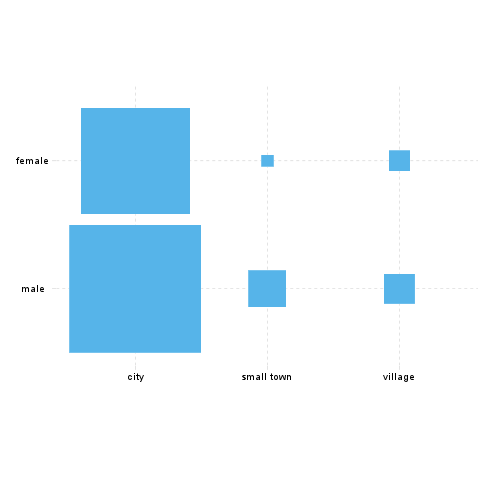## Description

Returning the Chi-squared test of two given variables with count, percentages and Pearson's residuals table.

### Variable description

Two variables specified:

• "email" ("Email usage") with 672 valid values and
• "dwell" ("Dwelling") with 662 valid values.

#### Introduction

Crosstables are applicable to show the frequencies of categorical variables in a matrix form, with a table view.

We will present four types of these crosstables. The first of them shows the exact numbers of the observations, ergo the number of the observations each of the variables' categories commonly have.

The second also shows the possessions each of these cells have, but not the exact numbers of the observations, rather the percentages of them from the total data.

The last two type of the crosstabs contain the so-called row and column percentages which demonstrate us the distribution of the frequencies if we concentrate only on one variable.

After that we present the tests with which we can investigate the possible relationships, associations between the variables, like Chi-squared test, Fisher Exact Test, Goodman and Kruskal's lambda.

In the last part there are some charts presented, with that one can visually observe the distribution of the frequencies.

### Counts

Counted values: "email" and "dwell" (continued below)
city small town village Missing
never 12 0 0 1
very rarely 30 1 3 2
rarely 41 3 1 1
sometimes 67 4 8 8
often 101 10 5 7
very often 88 5 5 10
always 226 9 7 17
Missing 34 1 1 1
Sum 599 33 30 47
Sum
never 13
very rarely 36
rarely 46
sometimes 87
often 123
very often 108
always 259
Missing 37
Sum 709

Most of the cases (226) can be found in "always-city" categories. Row-wise "always" holds the highest number of cases (259) while column-wise "city" has the utmost cases (599).

### Percentages

Total percentages: "email" and "dwell" (continued below)
city small town village Missing
never 1.69 0 0 0.14
very rarely 4.23 0.14 0.42 0.28
rarely 5.78 0.42 0.14 0.14
sometimes 9.45 0.56 1.13 1.13
often 14.25 1.41 0.71 0.99
very often 12.41 0.71 0.71 1.41
always 31.88 1.27 0.99 2.4
Missing 4.8 0.14 0.14 0.14
Sum 84.49 4.65 4.23 6.63
Sum
never 1.83
very rarely 5.08
rarely 6.49
sometimes 12.27
often 17.35
very often 15.23
always 36.53
Missing 5.22
Sum 100
Row percentages: "email" and "dwell"
city small town village Missing
never 92.31 0 0 7.69
very rarely 83.33 2.78 8.33 5.56
rarely 89.13 6.52 2.17 2.17
sometimes 77.01 4.6 9.2 9.2
often 82.11 8.13 4.07 5.69
very often 81.48 4.63 4.63 9.26
always 87.26 3.47 2.7 6.56
Missing 91.89 2.7 2.7 2.7
Sum 84.49 4.65 4.23 6.63
Column percentages: "email" and "dwell" (continued below)
city small town village Missing
never 2 0 0 2.13
very rarely 5.01 3.03 10 4.26
rarely 6.84 9.09 3.33 2.13
sometimes 11.19 12.12 26.67 17.02
often 16.86 30.3 16.67 14.89
very often 14.69 15.15 16.67 21.28
always 37.73 27.27 23.33 36.17
Missing 5.68 3.03 3.33 2.13
Sum
never 1.83
very rarely 5.08
rarely 6.49
sometimes 12.27
often 17.35
very often 15.23
always 36.53
Missing 5.22

### Tests of Independence

In the below tests for independece we assume that the row and column variables are independent of each other. If this null hypothesis would be rejected by the tests, then we can say that the assumption must have been wrong, so there is a good chance that the variables are associated.

#### Chi-squared test

One of the most widespread independence test is the Chi-squared test. While using that we have the alternative hypothesis, that two variables have an association between each other, in opposite of the null hypothesis that the variables are independent.

We use the cell frequencies from the crosstables to calculate the test statistic for that. The test statistic is based on the difference between this distribution and a theoretical distribution where the variables are independent of each other. The distribution of this test statistic follows a Chi-square distribution.

The test was invented by Karl Pearson in 1900. It should be noted that the Chi-squared test has the disadvantage that it is sensitive to the sample size.

##### Criteria

Before analyzing the result of the Chi-squared test, we have to check if our data meets some requirements. There are two widely used criteria which have to take into consideration, both of them are related to the so-called expected counts. These expected counts are calculated from the marginal distributions and show how the crosstabs would look like if there were complete independency between the variables. The Chi-squared test calculates how different are the observed cells from the expected ones.

The two criteria are:

• none of the expected cells could be lower than 1
• 80% of the expected cells have to be at least 5

Let's look at on expected values then:

city small town village
never 10.83 0.6134 0.5559
very rarely 30.69 1.738 1.575
rarely 40.62 2.3 2.085
sometimes 71.3 4.038 3.66
often 104.7 5.93 5.374
very often 88.45 5.01 4.54
always 218.4 12.37 11.21

We can see that the Chi-squared test met the requirements.

So now check the result of the test:

Pearson's Chi-squared test: table
Test statistic df P value
14.86 12 0.249

To decide if the null or the alternative hypothesis could be accepted we need to calculate the number of degrees of freedom. The degrees of freedom is easy to calculate, we substract one from the number of the categories of both the row and the coloumn variables and multiply them with each other.

To each degrees of freedom there is denoted a critical value. The result of the Chi-square test have to be lower than that value to be able to accept the nullhypothesis.

The requirements of the chi-squared test was not met, so Yates's correction for continuity applied. The approximation may be incorrect.

It seems that no real association can be pointed out between email and dwell by the Pearson's Chi-squared test (χ=14.86 at the degree of freedom being 12) at the significance level of 0.249.

##### References
• Fisher, R. A. (1922): On the interpretation of chi-square from contingency tables, and the calculation of P. Journal of the Royal Statistical Society 85 (1): 87-94.
• Fisher, R.A. (1954): Statistical Methods for Research Workers. Oliver and Boyd.

The residuals show the contribution to reject the null hypothesis at a cell level. An extremely high or low value indicates that the given cell had a major effect on the resulting chi-square, so thus helps understanding the association in the crosstable.

Residuals: "email" and "dwell"
city small town village
never 1.15 -0.81 -0.77
very rarely -0.41 -0.59 1.2
rarely 0.2 0.49 -0.8
sometimes -1.75 -0.02 2.49
often -1.28 1.9 -0.18
very often -0.17 0 0.24
always 2.1 -1.26 -1.64

Based on Pearson's residuals the following cells seems interesting (with values higher than 2 or lower than -2):

• "always - city"
• "sometimes - village"
##### References
• Snedecor, George W. and Cochran, William G. (1989): Statistical Methods. Iowa State University Press.
• Karl Pearson (1900): Philosophical Magazine, Series 5 50 (302): 157-175.

#### Fisher Exact Test

An other test to check the possible association/independence between two variables, is the Fisher exact test. This test is especially useful with small samples, but could be used with bigger datasets as well.

We have the advantage while using the Fisher's over the Chi-square test, that we could get an exact significance value not just a level of it, thus we can have an impression about the power of the test and the association.

The test was invented by, thus named after R.A. Fisher.

The test could not finish within resource limits.

### Charts

If one would like to investigate the relationships rather visually than in a crosstable form, there are several possibilities to do that.

###### Heat map

At first we can have a look at on the so-called heat map. This kind of chart uses the same amount of cells and a similar form as the crosstable does, but instead of the numbers there are colours to show which cell contains the most counts (or likewise the highest total percentages).

The darker colour is one cell painted, the most counts/the higher total percentage it has.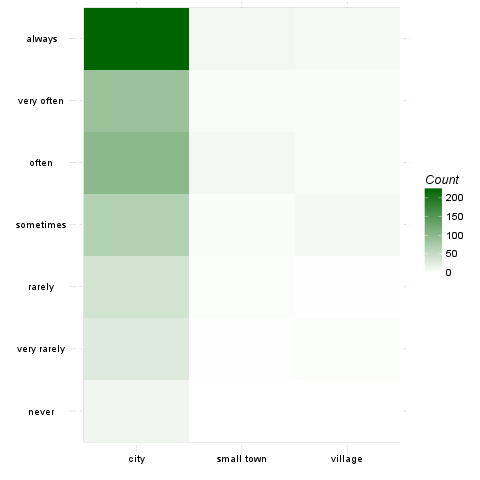There can be also shown the standardized adjusted residual of each cells: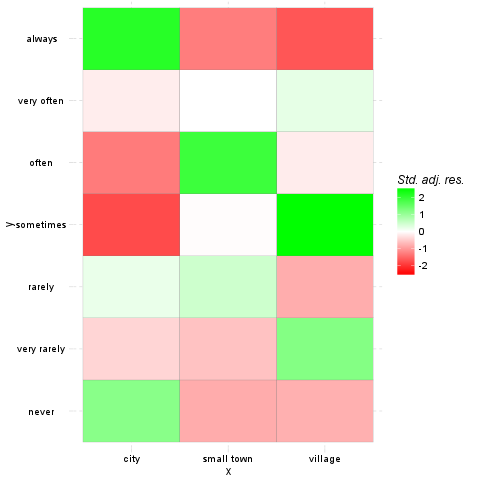###### Mosaic chart

In front of the heat map, on the mosaic charts, not only the colours are important. The size of the cells shows the amount of the counts one cell has.

The width on the axis of email determinate one side and the height on the axis of the dwell gives the final shape of the box. The box which demonstrates a cell from the hypothetic crosstable. We can see on the top of the chart which category from the dwell draw the boxes what kind of colour.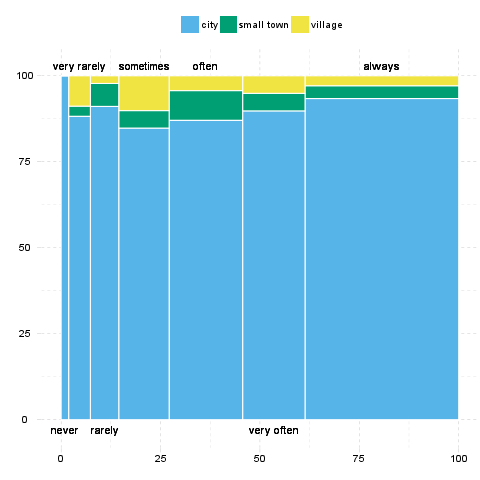###### Fluctuation diagram

At last but not least have a glance on the fluctuation diagram. Unlike the above two charts, here the colours does not have influence on the chart, but the sizes of the boxes, which obviously demonstrates here as well the cells of the crosstable.

The bigger are the boxes the higher are the numbers of the counts/the total percentages, which that boxes denote.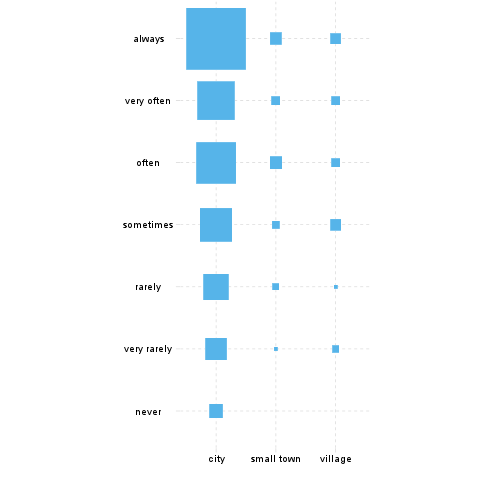This report was generated with R (3.0.1) and rapport (0.51) in 7.099 sec on x86_64-unknown-linux-gnu platform.Logo and design: © 2012 rapport Development Team | License (AGPL3) | Fork on GitHub | Styled with skeleton